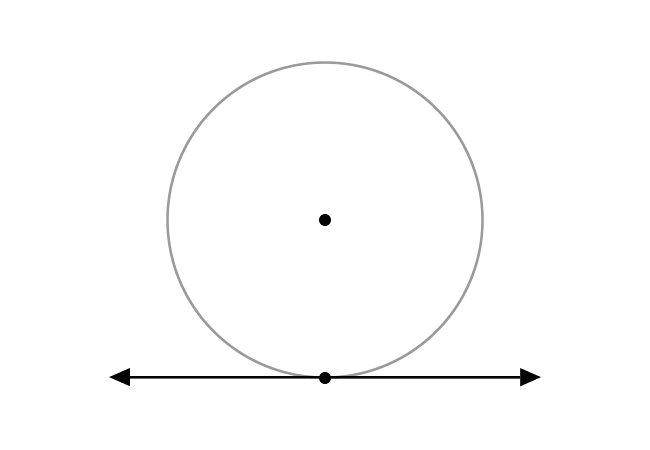Tangent of a circleDefinition

A straight line that passes through any point on the circumference of the circle is called a tangent of the circle.

A straight line can be passed through a circle in geometric system but in a special case, a straight line passes through a point of the circumference of the circle.

The straight line at that point is known as a tangent of the circle.

ExampleA circle consists of point $T$ as a point on its circumference.

A straight line $\overleftrightarrow{RS}$ is passed through the point $T$. So, the straight line $\overleftrightarrow{RS}$ at point $T$ is called a tangent of the circle geometrically.

Latest Math Topics
Latest Math Problems
Email subscription
Math Doubts is a best place to learn mathematics and from basics to advanced scientific level for students, teachers and researchers. Know more